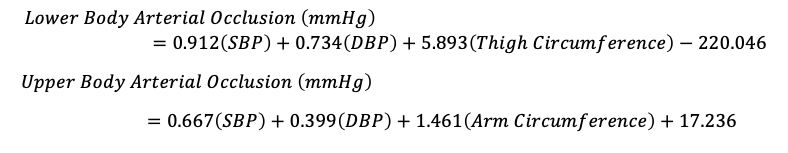# Blood Flow Restriction Without Doppler - Equation

Here at H+ Cuff, we suggest that clinical populations continue to use the "gold standard" external vascular doppler when calculating Limb Occlusion Pressure.

However, it can be completely safe and more practical to use a simple equation to calculate Limb Occlusion Pressure for healthy individuals performing Blood Flow Restriction.

It is important to note that this equation is specific for Wide Blood Flow Restriction Cuffs and should not be used with other more narrow Blood Flow Restriction Cuffs such as Bstrong BFR, EDGE Restriction Cuffs, or B3 Bands

*REMEMBER Wide Cuffs allow for lower pressures to be utilized!*It is important to note the following:
SBP = Systolic Blood Pressure
DBP = Diastolic Blood Pressure
Thigh/Arm Circumference = Measured in Centimeters

For example, if the subject is a healthy male with Blood Pressure of 120/80 and thigh circumference of 52 centimeters the lower body equation would look like the following:

= 0.912 (120) + .734 (80) + 5.893 (52) - 220.046
= 109.44 + 58.72 + 306.436 - 220.046
= 254.55 (This is 100% of your Limb Occlusion Pressure/Arterial Occlusion Pressure for the Lower Extremity)
= Since this is a lower extremity, BFR should be implemented at 80% or lower of LOP therefore the highest recommended pressure when implementing BFR for this person is 203.64 mmHg.

For Upper Extremity, a healthy male with Blood Pressure of 120/80 and arm circumference of 28 centimeters the upper body equation would look like the following:

= .667 (120) + .399 (80) +1.461 (28) + 17.236
= 80.04 + 31.92 + 40.908 + 17.236
= 170.104 This is 100% of your Limb Occlusion Pressure/Arterial Occlusion Pressure for the Upper Extremity)
= Since this is an upper extremity, BFR should be implemented at 50% or lower of LOP therefore the highest recommended pressure when implementing BFR for this person is 85.052 mmHg.

*Always feel free to email us at "TrainSmart@HPlusCuff.com" if you have any questions.